# How to Calculate and Solve for Length of Well Screen | Water Budget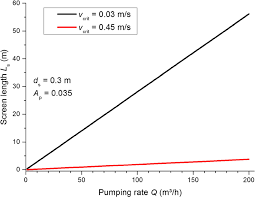The image above represents length of well screen.

To compute for length of well screen, three essential parameters are needed and these parameters are Maximum Expected Discharge Capacity of Well (Qo), Effective Open Area per Metre (Ao) and Entrance Velocity at the Screen (Ve).

The formula for calculating length of well screen:

L = Qo / AoVe

Where:

L = Length of Well Screen
Qo = Maximum Expected Discharge Capacity of Well
Ao = Effective Open Area per Metre
Ve = Entrance Velocity at the Screen

Let’s solve an example;
Find the length of well screen when the maximum expected discharge capacity of well is 12, the effective open area per metre is 24 and the entrance velocity at the screen is 18.

This implies that;

Qo = Maximum Expected Discharge Capacity of Well
Ao = Effective Open Area per Metre
Ve = Entrance Velocity at the Screen

L = Qo / AoVe
L = 12 / (24)(18)
L = 12 / 432
L = 0.027

Therefore, the length of well screen is 0.027.

Calculating the Maximum Expected Discharge Capacity of Well when the Length of Well Screen, the Effective Open Area per Metre and the Entrance Velocity at the Screen is Given.

Qo = L x (AoVe)

Where;

Qo = Maximum Expected Discharge Capacity of Well
L = Length of Well Screen
Ao = Effective Open Area per Metre
Ve = Entrance Velocity at the Screen

Let’s solve an example;
Find the maximum expected discharge capacity of well with a length of well screen as 21, effective open area per metre as 10 and entrance velocity at the screen as 5.

This implies that;

L = Length of Well Screen = 21
Ao = Effective Open Area per Metre = 10
Ve = Entrance Velocity at the Screen = 5

Qo = L x (AoVe)
Qo = 21 x (10 x 5)
Qo = 21 x 50
Qo = 1050

Therefore, the maximum expected discharge capacity of well is 1050.

Calculating the Effective Open Area per Metre when the Length of Well Screen, the Maximum Expected Discharge Capacity of Well and the Entrance Velocity at the Screen is Given.

Ao = (Qo / L) / Ve

Where;

Ao = Effective Open Area per Metre
L = Length of Well Screen
Qo = Maximum Expected Discharge Capacity of Well
Ve = Entrance Velocity at the Screen

Let’s solve an example;
Find the Effective Open area per metre when the length of well screen is 15, the maximum expected discharge capacity of well is 30 and the entrance velocity at the screen is 3.

This implies that;

L = Length of Well Screen = 15
Qo = Maximum Expected Discharge Capacity of Well = 30
Ve = Entrance Velocity at the Screen = 3

Ao = (Qo / L) / Ve
Ao = (30 / 15) / 3
Ao = 2 / 3
Ao = 0.67

Therefore, the effective open area per metre is 0.67.

Calculating the Entrance Velocity at the Screen when the Length of Well Screen, the Maximum Expected Discharge Capacity of Well and the Effective Open Area per Metre is Given.

Ve = (Qo / L) / Ao

Where;

Ve = Entrance Velocity at the Screen
L = Length of Well Screen
Qo = Maximum Expected Discharge Capacity of Well
Ao = Effective Open Area per Metre

Let’s solve an example;
Given that the length of well screen is 6, the maximum expected discharge capacity of well is 24 and the effective open area per metre is 2. Find the entrance velocity at the screen?

This implies that;

L = Length of Well Screen = 6
Qo = Maximum Expected Discharge Capacity of Well = 24
Ao = Effective Open Area per Metre = 2

Ve = (Qo / L) / Ao
Ve = (24 / 6) / 2
Ve = 4 / 2
Ve = 2

Therefore, the entrance velocity is 2.

Nickzom Calculator – The Calculator Encyclopedia is capable of calculating the length of well screen.

To get the answer and workings of the length of well screen using the Nickzom Calculator – The Calculator Encyclopedia. First, you need to obtain the app.

You can get this app via any of these means:

To get access to the professional version via web, you need to register and subscribe for NGN 1,500 per annum to have utter access to all functionalities.
You can also try the demo version via https://www.nickzom.org/calculator

Apple (Paid) – https://itunes.apple.com/us/app/nickzom-calculator/id1331162702?mt=8
Once, you have obtained the calculator encyclopedia app, proceed to the Calculator Map, then click on Agricultural under Engineering.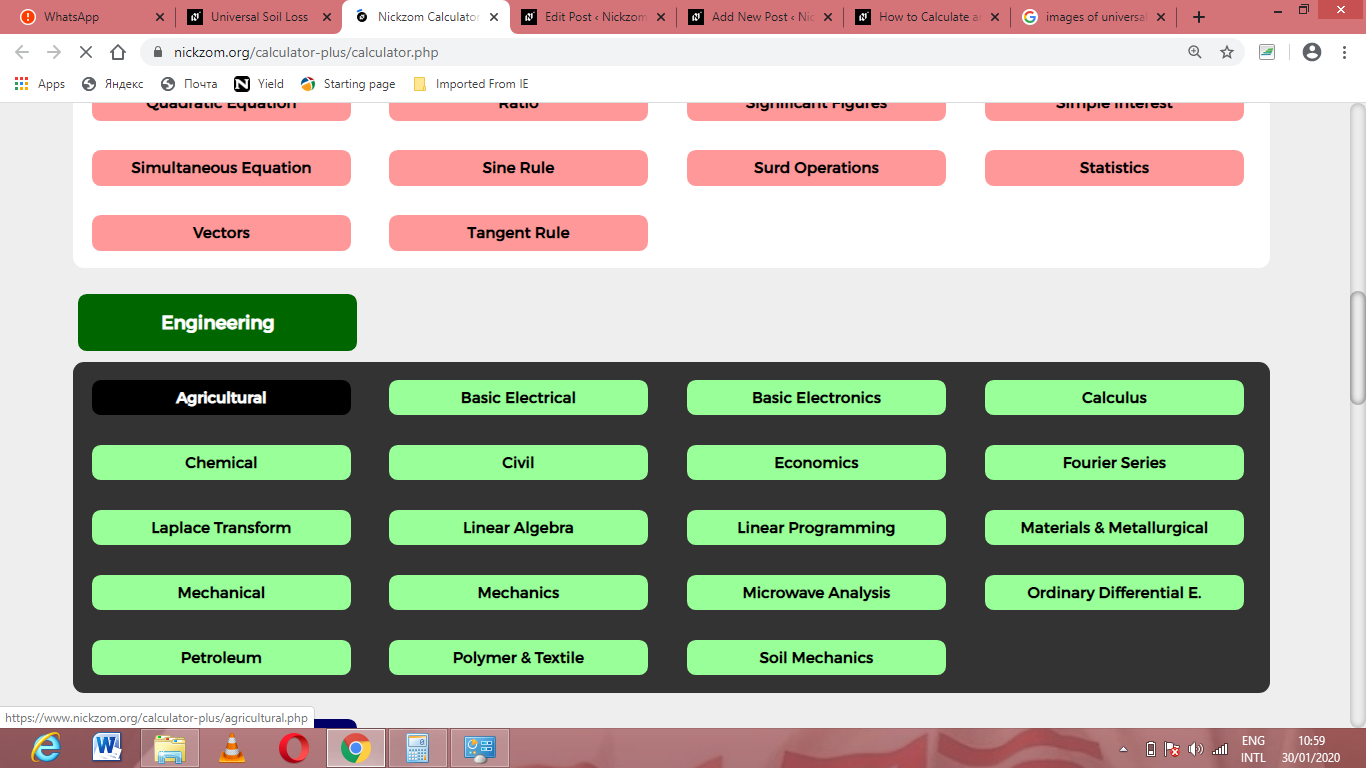Now, Click on Water Budget under Agricultural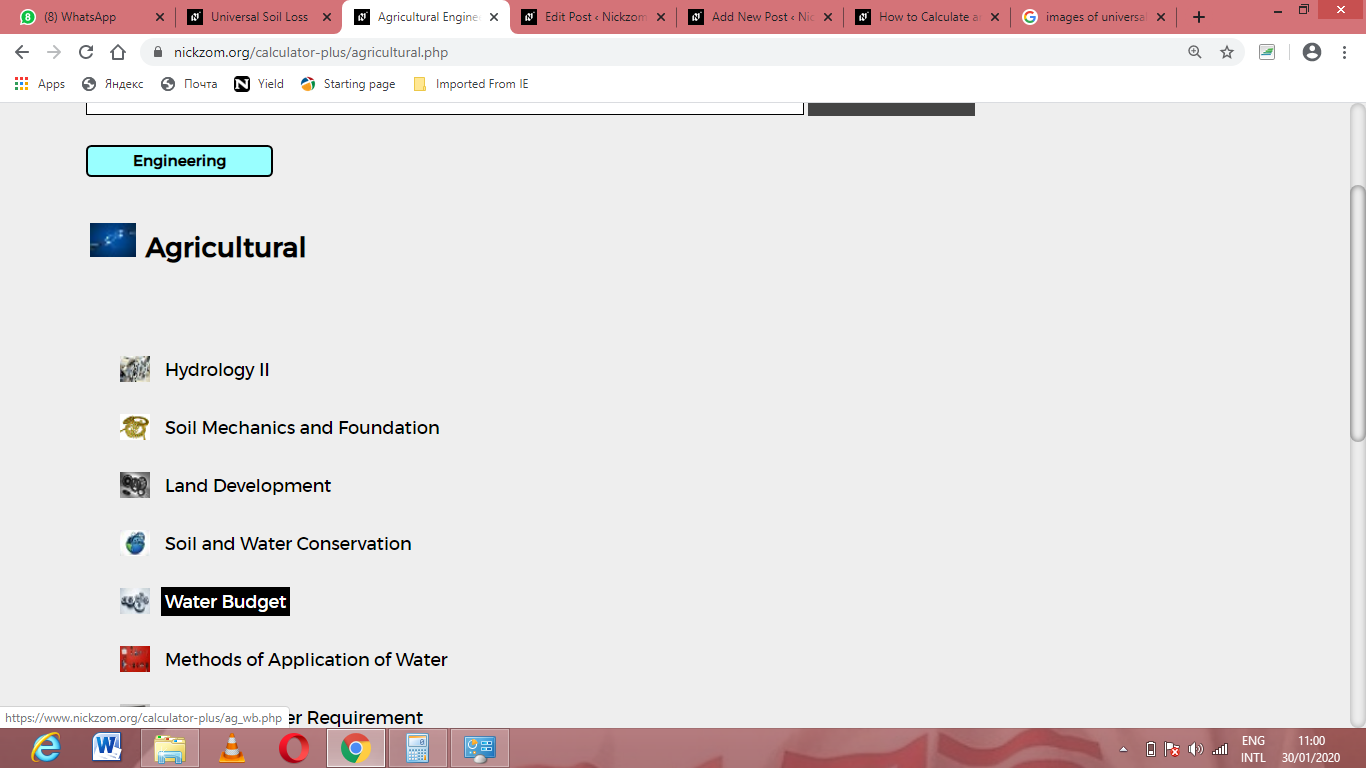Now, Click on Length of Well Screen under Water Budget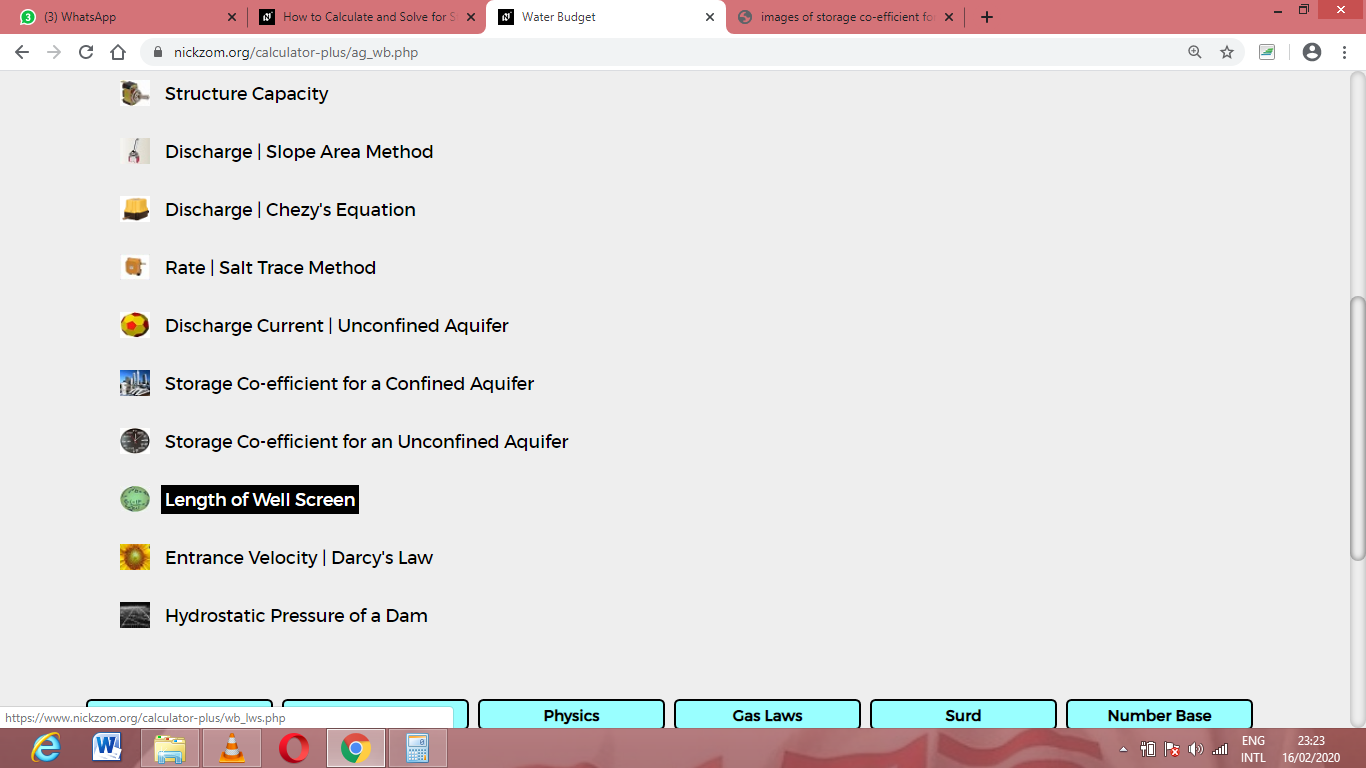The screenshot below displays the page or activity to enter your values, to get the answer for the length of well screen according to the respective parameters which are the Maximum Expected Discharge Capacity of Well (Qo), Effective Open Area per Metre (Ao) and Entrance Velocity at the Screen (Ve).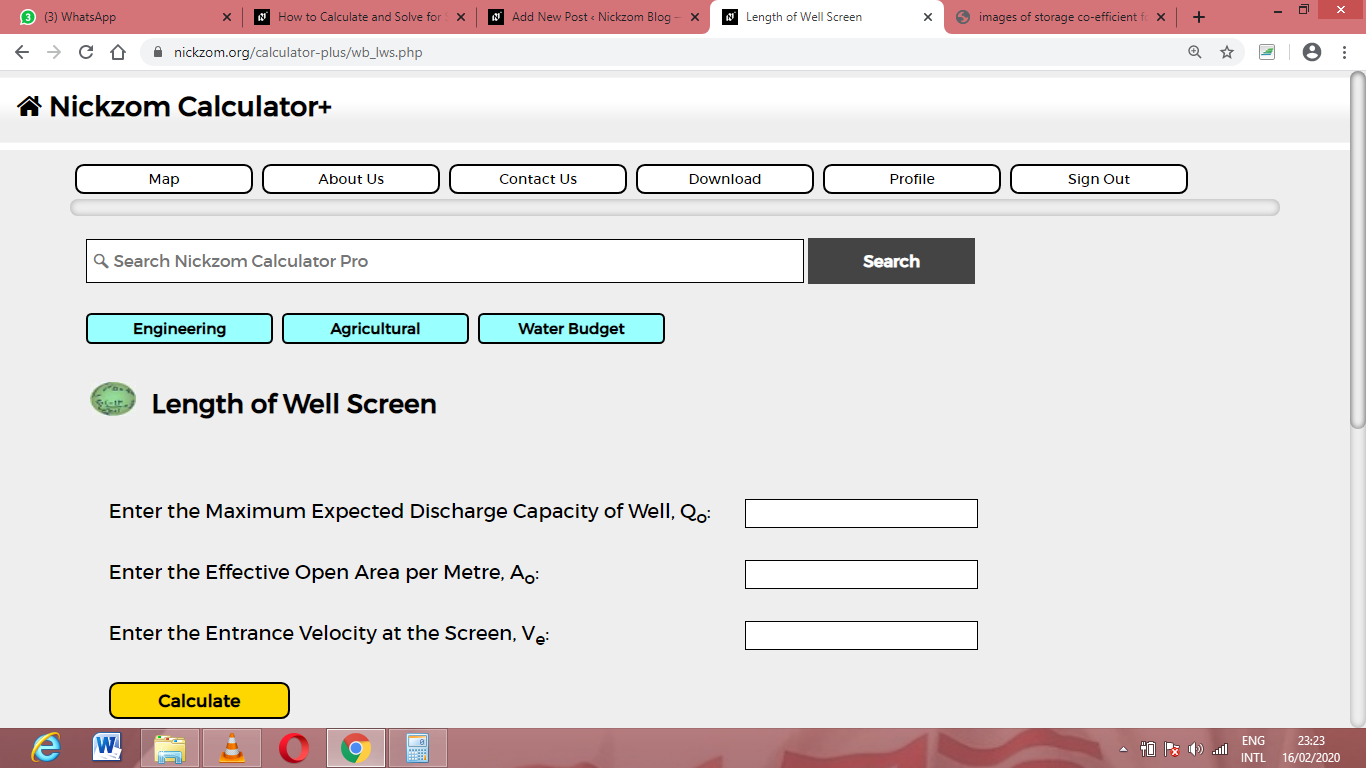Now, enter the values appropriately and accordingly for the parameters as required by the Maximum Expected Discharge Capacity of Well (Qo) is 12, Effective Open Area per Metre (Ao) is 24 and Entrance Velocity at the Screen (Ve) is 18.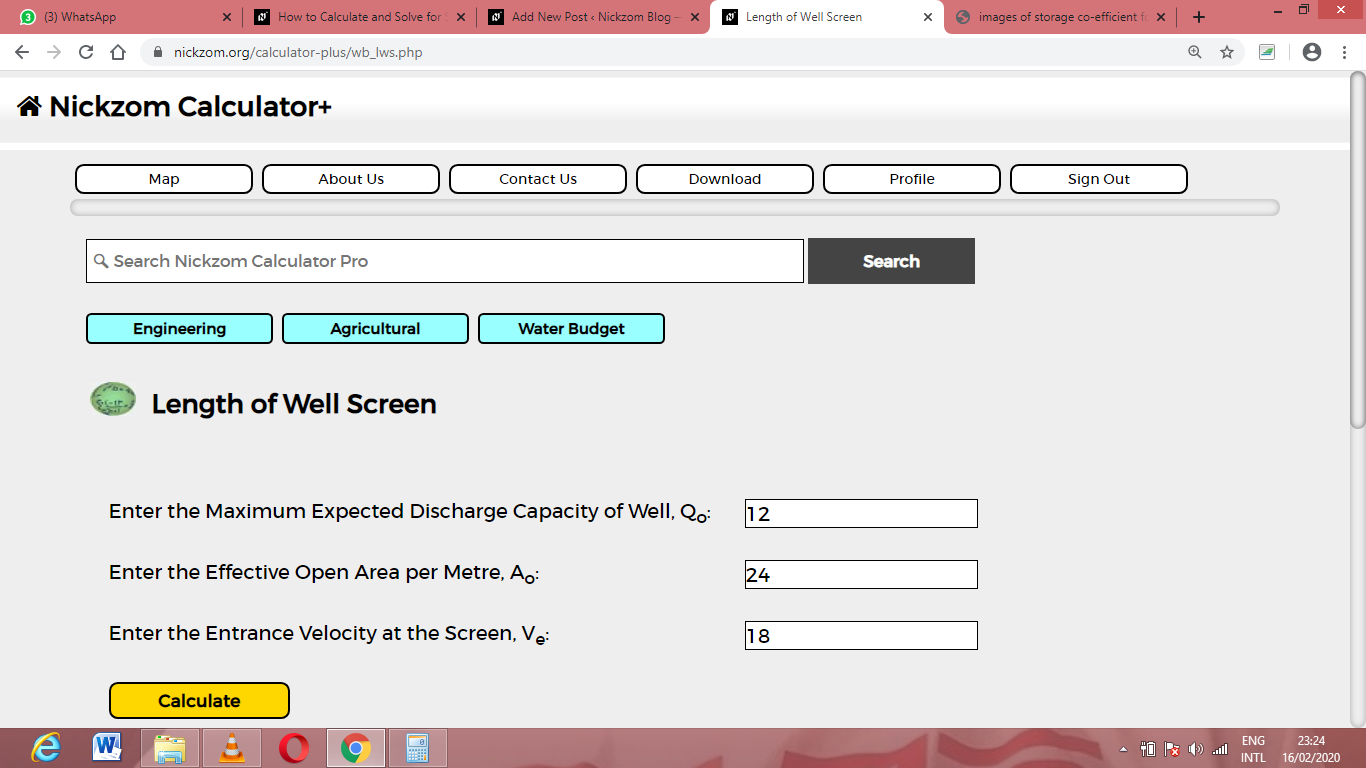Finally, Click on Calculate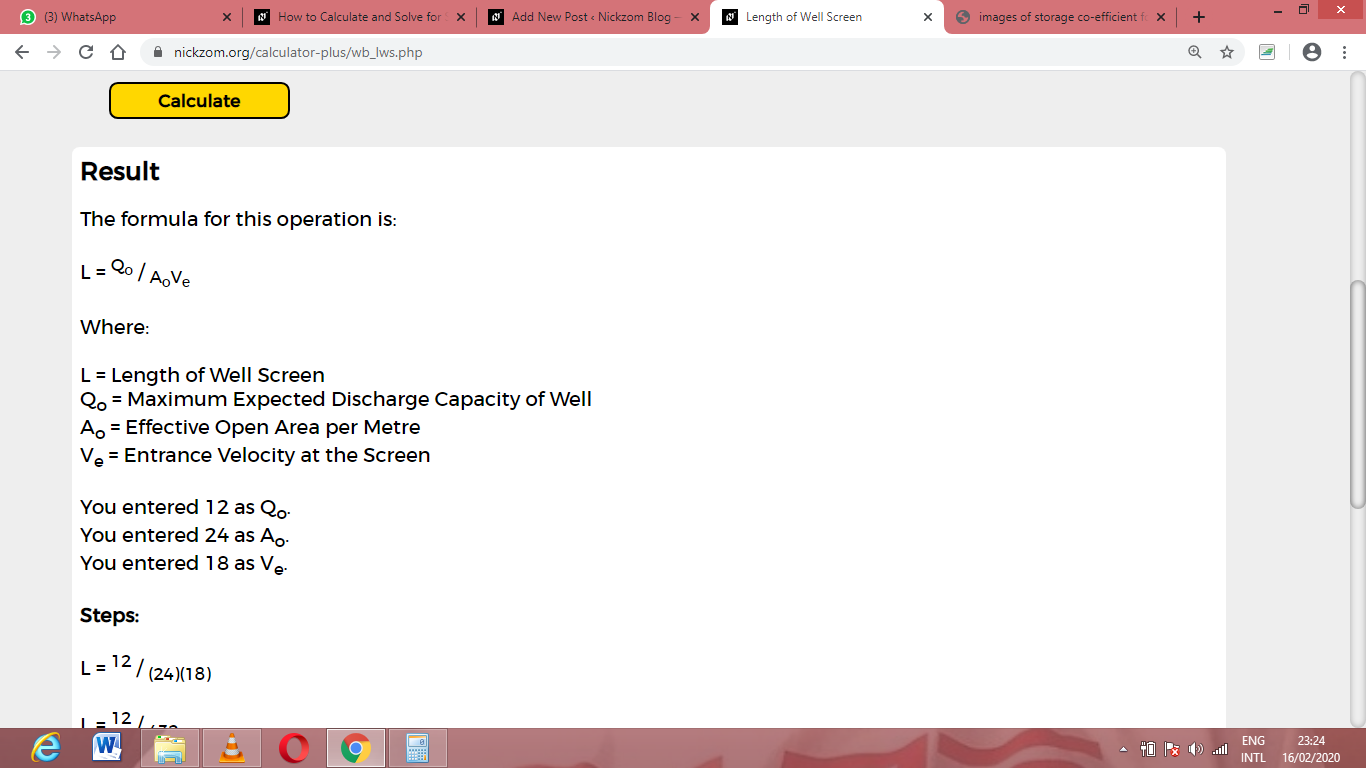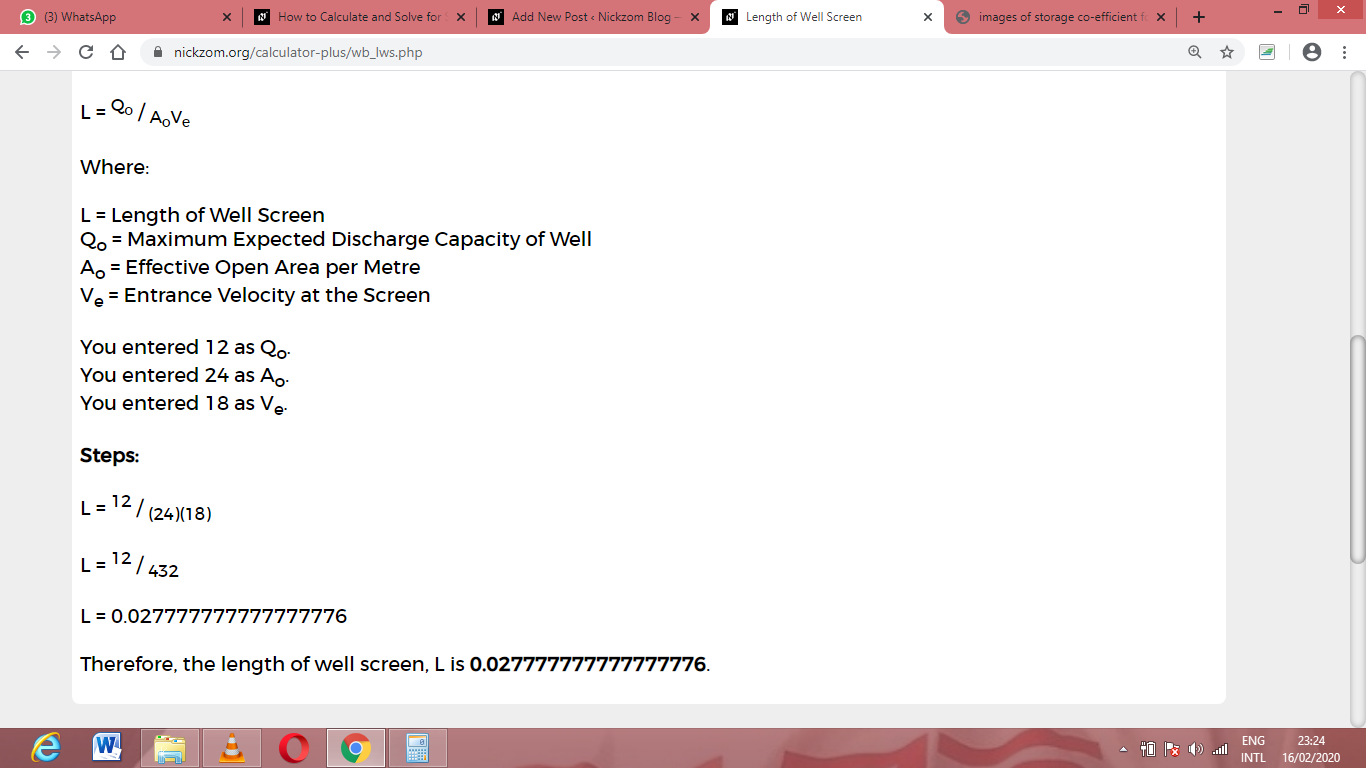As you can see from the screenshot above, Nickzom Calculator– The Calculator Encyclopedia solves for the length of well screen and presents the formula, workings and steps too.